# Optimal Energy Tracking in a Solar Power System Utilizing Synthetic Neural Network

Optimal Energy Tracking in a Solar Power System Utilizing Synthetic Neural Network
Department of Electrical Engineering, Badji Mokhtar University of Annaba, Annaba 23000, Algeria

Department of Computer Science, Chadli Bendjedid University of El Taref, El Taref 36000, Algeria

Corresponding Author Email:
amel.ourici@hotmail.com
Page:
15-20
|
DOI:
https://doi.org/10.18280/ejee.250103
15 December 2022
|
Revised:
16 January 2023
|
Accepted:
23 January 2023
|
Available online:
28 Feburary 2023
| Citation

OPEN ACCESS

Abstract:

In this particular study, an artificial neural network (ANN) was utilized to effectively extract the maximum power from a photovoltaic (PV) installation. The primary objective was to assess the performance of the ANN in comparison to the commonly used perturb and observe (P&O) technique, especially when faced with varying climatic conditions.

The results of the study unequivocally demonstrated the superiority of artificial intelligence, specifically the ANN, in enhancing the optimization of PV power. When compared to the traditional P&O technique, the ANN consistently outperformed it in terms of accurately tracking the maximum power point under different climatic conditions. This highlights the remarkable capabilities of artificial intelligence in improving the efficiency and performance of PV systems.

Keywords:

solar panel, maximum power, artificial neural network, quadratic error

1. Introduction

Renewable energies offer numerous advantages over fossil fuels in terms of both environmental impact and greenhouse gas emissions. Firstly, renewable energy sources such as solar, wind, hydro, and geothermal power are inherently sustainable and inexhaustible, unlike fossil fuels that are finite and non-renewable. This ensures a long-term and reliable energy supply for future generations.

Transitioning to renewable energy also brings economic benefits. It fosters job creation and local economic growth by supporting the development, manufacturing, installation, and maintenance of renewable energy systems. Additionally, renewable energy investments often lead to reduced energy costs in the long run, as the fuel sources are abundant and freely available.

In this context, photovoltaic systems, which typically include PV solar generators, inverters, MPPT controllers, and loads (DC or AC), provide a highly competitive solution.

Photovoltaic (PV) systems have emerged as one of the most promising renewable energy sources, with their ability to produce electricity directly from sunlight. However, the performance of these systems depends on many factors, such as temperature, irradiance, and shading, which can affect their efficiency and output power. Peak power point tracking (MPPT) is a widely used technique that aims to find the operating point at which a PV system produces maximum power output. Traditional MPPT methods, such as perturbation and observation (P&O) and increasing conductivity (IC), are simple and low-cost but can be slow and less accurate in tracking the maximum power point.

Perturb and observe (P&O) and ascending conductivity techniques have obvious shortcomings, such as poor tracking performance during rapidly changing solar irradiance and oscillations around the power point. Maxima. The smart techniques include Fuzzy Logic Controller (FLC), Artificial Neural Network (ANN), Particle Swarm Optimization (PSO), Genetic Algorithm (GA). These algorithms have many advantages, such as low oscillation around the peak power point and fast tracking response to changing conditions.

Recently, the use of artificial intelligence (AI) techniques, such as neural networks (NN), has shown great potential for improving the accuracy and efficiency of MPPT in PV systems. Moreover, neural networks are a type of machine learning algorithm that can learn from data and make predictions based on that data. They can be trained to predict the maximum power point based on the input parameters, such as temperature and irradiance, and adjust the PV system's operating point accordingly. Several studies have shown the effectiveness of using NN-based MPPT algorithms in PV systems. For example, Jyothy and Sindhu  provided a comprehensive review of the use of NN for MPPT in PV systems, highlighting the advantages and limitations of various NN-based techniques. Villegas-Mier et al.  reviewed recent advances in AI-based MPPT, including NN-based algorithms, and discussed their performance and challenges. Chaabene et al.  proposed an NN-based fractional-ordered sliding mode control algorithm for MPPT in PV systems., which showed improved performance compared to traditional methods. However, other researchers have combined NN with other optimization algorithms, such as gravitational search algorithm (GSA) , particle swarm optimization (PSO) , and cuckoo search optimization , to enhance the accuracy and convergence speed of MPPT in PV systems. Takruri et al.  developed an NN-based MPPT algorithm that can adapt to variable irradiance and temperature conditions, improving the robustness and stability of the PV system. In summary, NN-based MPPT algorithms have shown great potential for improving the performance and efficiency of PV systems. However, there are still some challenges that need to be addressed, such as the need for large amounts of training data, the complexity of NN models, and the computational cost. Nevertheless, with the growing demand for clean energy and the advances in AI and machine learning, the use of NN in PV systems is likely to become more widespread in the future.

This article supports research in this section, which is extracting maximum power using an artificial neural network.

In this paper, we present a peak power monitoring study for photovoltaic energy Processing line. We offer a comparison between two peak power monitoring techniques; Perturb & Observe (P&O) is the first method compared to the second method using artificial neural network (ANN). Both methods are designed, modeled and simulated in the MATLAB/Simulink environment. The simulation results are discussed regarding the performance and constraints of these two algorithms.

2. Photovoltaic Generator Studied

The model studied was used for the perturb and observe method, in order to be able to compare these results later with the maximum power track method with the artificial neural networks method.

## 1.png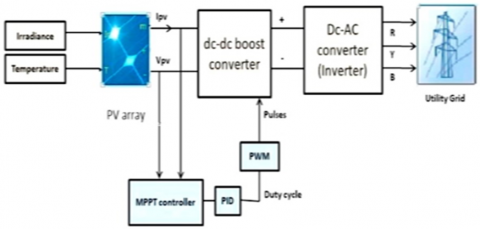Figure 1. Scheme of photovoltaic generator studied

We worked on a 100 kw PV array made from modules of the type SunPower SPR-305-WHT, that consists of 96 cells, number of modules connected in series per string is 5, and number of parallel strings is 66. This PV array is associated with a boost chopper, with a perturb and observe maximum power point tracking control, as a first approach., which is illustrate on Figure 1.

Figure 1 illustrates the general model with which the perturb and observe method was simulated.

The boost converter serves as an interface between photovoltaic generator and load to increase voltage at the output of the PV array. It mainly consists of an inductor, a transistor switch, a diode and filter capacitor.

A real array consists of multiple connected PV cells, and to observe properties at the terminals of the PV array, additional parameters must be included in the basic equations [8, 9].

$I=I_{\mathrm{pV}}-I_0\left[\exp \left(\frac{V+R_5 I}{V_t a}\right)-1\right]-\frac{V+R_5 I}{R_P}$       (1)

The maximum output will fluctuate due to changes in ambient temperature and amount of solar radiation. Real-time peak power point trackers are an integral part of a solar system because the maximum power available from a solar system varies continuously with atmospheric conditions. Maximum power point tracking MPPT systems proposed in the literature  can be classified into three different categories .

The direct method, the intelligent method, and the indirect method.

The direct method is also called truth-seeking method (MPPT). It is searched by disturbances going on at the operating point of the PV generator. In this category, scheme perturbation and observation P&O , hill-climbing HC , and incremental conductivity INC  are widely applied to PV systems. In P&O method, the working voltage of PV array is changed to achieve MPPT. Similar to the P&O.

First, we simulated the perturb and observe method for our system, imposing the variations in irradiation and temperature, shown on the Figure 2, irradiation and ambient temperature, and we got the results shown on Figure 3, which are the output power of the PV array and the duty cycle.

The P&O technique continuously increases or decreases the voltage across the PV array by a small amount until the peak power point is reached and compares the trend of the output power with the trend of the noise. Thus, the disturbance will continue in the same direction if the output power increases and reverse if it decreases. the algorithm continues the same way.

## 2.png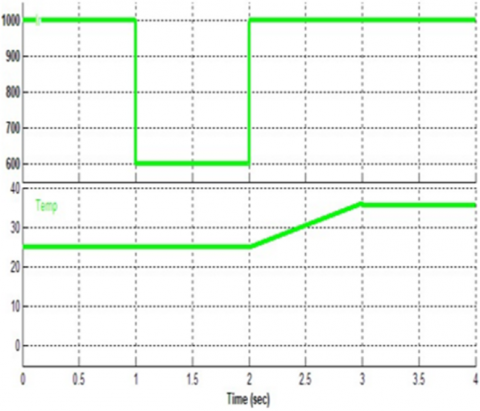Figure 2. Required variations in irradiation and temperature

## 3.pngFigure 3. Output power of the PV array and the duty cycle with the P&O method

3. Design of the Adapted Artificial Neural Network

Neural networks work by distributing the values of variables in automata (neurons). These units are responsible for combining their information to determine the value of the discrimination parameter. It is from the connection of these units to each other that the RNA’s ability to discriminate emerges. Each neuron receives numerical information from neighbouring neurons; each of these values is associated with a weight representative of the strength of the connection. Each neuron performs a calculation locally, the result of which is then transmitted to the avalous neurons.

## 4.png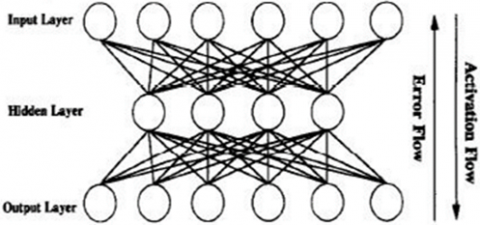Figure 4. A fully connected backpropagation network, with the direction of activation and error flow indicated

The proven potential of machine learning techniques in pattern matching and computer vision has led researchers to use these techniques to predict the efficiency of solar cells [15-22]. The research work performed to date demonstrates the applications of these techniques for optimal efficiency prediction, best-suited design and materials for fabricating dye-sensitive solar cells (DSSCs). The network we used, is a fully connected backpropagation one as shown on the Figure 4.

The general objective of an artificial neural network is to find the configuration of the connection weights between neurons so that it associates with each input configuration, an adequate response. The use of an NAS is done in two stages. First a learning phase which is responsible for establishing values for each of the network connections, then a phase of use proper, where we present to the network an input and where it tells us in return its "calculated" output.

The process of designing our neural network can be summarized in these steps:

-The collection of a database: this data constitutes the input of the neural network, and therefore it determines both the size of the network and the performance of the system, in our study we have only one input variable which is the power of the photovoltaic generator and a single output which is the cyclic ratio' D', we collected these data from the solar panel simulation with perturb and observe control. The purpose of this step is to gather enough data to build a representative database, which will be used for learning and testing the neural network. This database constitutes the input of the neural network, and therefore it determines both the size of the network (and therefore the simulation time), and the performance of the system (generalization capacity).

-We built a network of multi-layered neurons with:

An input layer containing 2 neurons, a hidden layer containing 15 neurons, and an output layer containing 1 neuron.

We used sigmoid functions for the input, output and hidden layer transfer functions.

- Training neural networks based on learning and validation. I ran machine learning until I got a very small squared error.

- Measure neural network performance on a test basis. With all this data we were able to build a network of neurons under matlab simulink and theoretically speaking, this network of neurons was made on the basis of these equations.

Network learning by backstabbing involves three steps:

- Enable propagation or feedforward of drive model from inputs until the exit,

- Calculate and reverse propagate the associated error,

a- The yield of the idle layer

$y_i=f_j^c\left(\sum_{t=1}^n w_{j i}^c x_t+b_j^c\right)$       (2)

b- The yields of the yield layer

$o_k=f_k^s\left(\sum_{j=1}^m w_{k j}^s y_j+b_k^s\right)$        (3)

c- The mistake terms of the yield units

$\delta_k^s=(t k-o k) \int_k^{s^{\prime}}\left(\sum_{t-1}^m w_{k j}^5 y j+b_k^*\right)$       (4)

e- Weight and predisposition alteration of the yield layer

$\begin{gathered}w_{k j}^s(t+1)=w_{k j}^s(t)+\eta \delta_k^s y_j \\ b_k(t+1)=b_k(t)+\eta \delta_k\end{gathered}$      (5)

## 5.pngFigure 5. Neural network built with MATLAB

The input of the neural network is the power released by the photovoltaic generator, and the output is the cyclic ratio D (obtained during the previous simulation).We performed a learning on 100 data using Matlab software as shown on Figure 5, until a very small quadratic error , show in Figure 6.

Figure 4 shows the general diagram of a backpropagation network used in the simulation.

## 6.png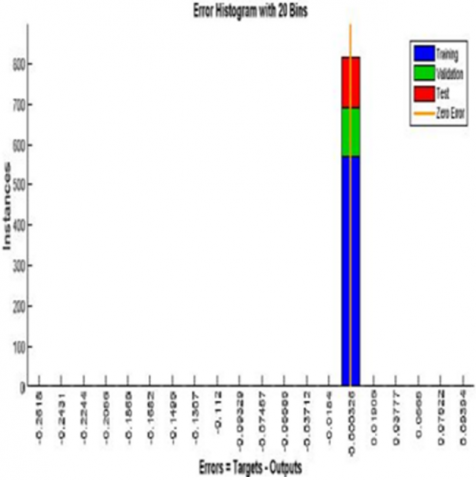The connection weight values are first randomly created and the system tries in successive iterations to obtain data modeling. At each stage, an input is presented to the network, it propagates these values to the output neurons. This calculated output is compared with the expected response and the system modifies the weights accordingly. This connection alteration is obtained by the error gradient backscatter algorithm. This calculation is responsible for backscattering the errors found on the outputs. In theory, one can never be sure that this algorithm will eventually determine a suitable weight set for all input-output pairs. In practice, we do not build a single RNA, but several models by playing on the parameters of this algorithm, and by seeking to obtain a model that best fits the data.

The development of a neural network, whatever its architecture, requires some experience [23-25]. This is why collaboration with specialists is the most desirable approach, whether to supervise the use of simple software such as SPSS©, MATLAB©, STATISTICA© which offer neural networks modules, or for developing RNA from more sophisticated software. As the application of artificial neural networks is booming in all areas of research, this interdisciplinarity is easily conceivable. Artificial neural networks are therefore a data processing tool which, like traditional statistics, can be applied in several fields .

Figure 5 illustrates the neural network built with MATLAB Simulink.

The next step is to minimize the quadratic error as much as possible.

The generalisation error depends on three parameters: the number of examples used for learning, the complexity of the underlying problem and the network architecture. Statistical approaches are one of the major areas of investigation for optimizing the learning performance of neural networks [27, 28].

Methods that evaluate generalization error are generally based on the partition of available data into three subsets. The first subset called learning set is used to optimize network weights for a given architecture. The second subset, called a validation set, allows you to compare several network architectures and select the best one.

The second set also makes it possible to monitor and measure the generalisation of the network during learning. Finally, the third subset called the test set is used to estimate the network generalization error determined from the learning and validation sets.

Due to the need to calculate the mean square error and variance of the mean square error after each weight change, the calculation time can become very important. Indeed, for each presentation of an example, it is necessary to carry out a number of propagations and a backscatter. Also, in order to reduce the computation time, we will use the algorithm mentioned above which, as we will see later, allows to introduce a stronger constraint on the control of the form of the error distribution and to add stability to the learning process.

In this test, the ANN technique was able to quickly track MPP under rapidly changing solar irradiance, while P&O did not track MPP under rapidly changing irradiance. In addition, the ANN has small fluctuations around the maximum power point, while the P&O technique has high fluctuations around the maximum power point MPP.

4. Results Analysis

Despite the same variations in irradiation and ambient temperature, the results of the simulation of the power output of the photovoltaic generator, Figures 3 and 7, clearly show, that the maximum power has been reached by the artificial neural networks method, which is 100 kw, which minimizes excessive power loss. this was not achieved with the perturb and observe method, where it can be seen that the power shown in the Figure 3 could not reach the maximum power of the installation, and this with the same variations in irradiation and temperature applied to the neural networks method. The output voltage of the PV array shown on Figure 8 is also kept constant by the latter method. We can say that the neural networks method is very robust compared to the Perturb and observe method, and since the power loss is very minimal for the neural networks method, it can be said that the solar generator yield is much better than the P&O method.

## 7.png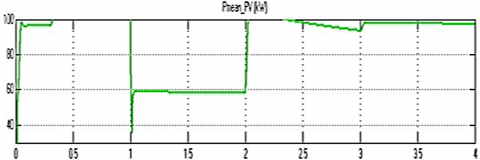Figure 7. Output power of PV panel with ANN method

## 8.png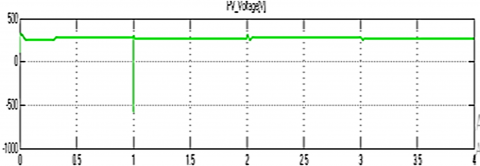Figure 8. Output PV voltage with ANN method

5. Conclusion

In this article, we simulated a PV array of 100 Kw, with MPPT control, with conventional P&O mepido, then with a neural network. The simulation results obtained are very promising and in general, the performance of the MPPT based on artificial neural network ANN, in terms of stability, accuracy and speed in the continuation of the maximum power point MPP are much better than the controller based on conventional MPPT method (P&O). In conclusion, the use of neural networks for maximum power tracking (MPT) in solar panels has shown promising results. Neural networks can effectively learn the complex nonlinear relationships between the input parameters, such as irradiance and temperature, and the output parameter, which is the maximum power point voltage and current. The ability of neural networks to handle noisy and incomplete data, as well as their adaptability to changing environmental conditions, makes them a suitable solution for MPT in solar panels. Several studies have demonstrated the effectiveness of neural networks for MPT in solar panels, with some achieving higher accuracy and efficiency compared to traditional techniques such as perturbations and observed and increased conductivity. Moreover, the use of deep learning techniques, such as convolutional neural networks and recurrent neural networks, has shown even more promising results. However, the use of neural networks for MPT in solar panels still requires further research and development.

In summary, this section developed an artificial NN model for MPPT in the PV system. The proposed NN was trained on operational data and found to effectively track the maximum power point under varying conditions. Although the model shows promise for improving MPPT performance, further testing with larger datasets is required to validate its effectiveness.

Nomenclature
 IPV the PV current I0 saturation current of photovoltaic generator Vt thermal voltage of the PV Ns connected cells in series Rs equivalent series resistance Rp equivalent parallel resistance Yi The yield of the idle layer ok The yields of the yield layer δk The mistake terms of the yield units wk weight of the yield layer bk bias adjustment of the yield layer
References

 Jyothy, L.P.N., Sindhu, M.R. (2018). An artificial neural network based MPPT algorithm for solar PV system. In 2018 4th International Conference on Electrical Energy Systems (ICEES), Chennai, India, pp. 375-380. https://doi.org/10.1109/ICEES.2018.8443277

 Villegas-Mier, C.G., Rodriguez-Resendiz, J., Álvarez-Alvarado, J.M., Rodriguez-Resendiz, H., Herrera-Navarro, A.M., Rodríguez-Abreo, O. (2021). Artificial neural networks in MPPT algorithms for optimization of photovoltaic power systems: A review. Micromachines, 12(10): 1260. https://doi.org/10.3390/mi12101260

 Dobreva, P., van Dyk, E.E., Chaari, S., Vorster, F.J. (2020). New approach to evaluating predictive models of photovoltaic systems. Solar Energy, 204: 134-143. https://doi.org/10.1016/j.solener.2020.04.028

 Sahraoui, H., Mellah, H., Drid, S., Chrifi-Alaoui, L. (2021). Adaptive maximum power point tracking using neural networks for a photovoltaic systems according grid. Electrical Engineering & Electromechanics, (5): 57-66. https://doi.org/10.20998/2074-272X.2021.5.08

 Priscila, D., Al. (2020). Analysis of solar and wind energy installations at electric vehicle charging stations in a region in Brazil and their impact on pricing using an optimized sale price model. International Journal of Energy Research, 45(5). https://doi.org/10.1002/er.6269

 Eltamaly, A.M. (2021). An improved cuckoo search algorithm for maximum power point tracking of photovoltaic systems under partial shading conditions. Energies, 14(4): 953. https://doi.org/10.3390/en14040953

 Takruri, M., Farhat, M., Barambones, O., Ramos-Hernanz, J.A., Turkieh, M.J., Badawi, M., AlZoubi, H., Abdus Sakur, M. (2020). Maximum power point tracking of pv system based on machine learning. Energies, 13(3): 692. https://doi.org/10.3390/en13030692

 Villalva, M.G., Gazoli, J.R., Filho, E.R. (2009). Comprehensive approach to modeling and simulation of photovoltaic arrays. IEEE Transactions on Power Electronics, 24(5): 1198-1208. https://doi.org/10.1109/TPEL.2009.2013862

 Lasnier, F., Ang, T.G. (1990). Photovoltaic Engineering Handbook, Adam Hilger, New York, NY, USA.

 Esram, T., Chapman, P.L. (2007). Comparison of photovoltaic array maximum power point tracking techniques. IEEE Transactions on Energy Conversion, 22(2): 439-449.

 Salas, V., Olías, E., Barrado, A., Lázaro, A. (2006). Review of the maximum power point tracking algorithms for stand-alone photovoltaic systems. Solar Energy Materials and Solar Cells, 90(11): 1555-1578. https://doi.org/10.1016/j.solmat.2005.10.023

 Wasynczuk, O. (1983). Dynamic behavior of a class of photovoltaic power systems. IEEE Transactions on Power Apparatus and Systems, 102(9): 3031-3037.

 Koutroulis, E., Kalaitzakis, K., Voulgaris, N.C. (2001). Development of a microcontroller-based, photovoltaic maximum power point tracking control system. IEEE Transactions on Power Electronics, 16(1): 46-54.

 Hussein, K.H., Muta, I., Hoshino, T., Osakada, M. (1995). Maximum photovoltaic power tracking: An algorithm for rapidly changing atmospheric conditions. IEEE Proceedings: Generation, Transmission and Distribution, 142(1): 59-64.

 Sahu, H., Rao, W., Troisi, A., Ma, H. (2018). Toward Predicting efficiency of organic solar cells via machine learning and improved descriptors. Advanced Energy Materials, 8(24). https://doi.org/10.1002/aenm.201801032

 Meftahi, N., Klymenko, M., Christofferson, A.J., Gao, F., Brédas, J.L. (2020). Machine learning property prediction for organic photovoltaic devices. npj Computational Materials, 6(1): 166. https://doi.org/10.1038/s41524-020-00429-w

 Lee, C.H., Yang, H.C., Ye, G.B. (2021). Predicting the performance of solar power generation using deep learning methods. Applied Sciences, 11(15): 6887. https://doi.org/10.3390/app11156887

 Alam, M.S., Al-Ismail, F.S., Hossain, M.S., Rahman, S.M. (2023). Ensemble machine-learning models for accurate prediction of solar irradiation in Bangladesh. Processes, 11(3): 908. https://doi.org/10.3390/pr11030908

 Sun, W.B. et al. (2019). Machine learning–assisted molecular design and efficiency prediction for high-performance organic photovoltaic materials. Science Advances. https://doi.org/10.1126/sciadv.aay4275

 Alwadai, N., Khan, S.U.D., Elqahtani, Z.M., Ud-Din Khan, S. (2022). Machine learning assisted prediction of power conversion efficiency of all-small molecule organic solar cells: A data visualization and statistical analysis. Molecules, 27(18): 5905. https://doi.org/10.3390/molecules27185905.

 Yang, B., Yu, T., Zhang, X., Li, H., Shu, H., Sang, Y., Jiang, L. (2019). Dynamic leader based collective intelligence for maximum power point tracking of PV systems affected by partial shading condition. Energy Conversion and Management, 179: 286-303. https://doi.org/10.1016/j.enconman.2018.10.074

 Armghan, H., Ahmad, I., Armghan, A., Khan, S., Arsalan, M., Naghmash. (2018). Backstepping based non-linear control for maximum power point tracking in photovoltaic system. Solar Energy, 159: 134-141. https://doi.org/10.1016/j.solener.2017.10.062

 Haq, I.U., Khan, Q., Khan, I., Akmeliawati, R., Nisar, K. S., Khan, I. (2020). Maximum power extraction strategy for variable speed wind turbine system via neuro-adaptive generalized global sliding mode controller. IEEE Access, 8: 128536-128547.

 Abo-Sennah, A., El-Dabah, M.A., El-Biomey Mansour, A. (2021). Maximum power point tracking techniques for photovoltaic systems: A comparative study. International Journal of Electrical and Computer Engineering (IJECE), 11(1): 2088-8708. http://dx.doi.org/10.11591/ijece.v11i1.pp57-73

 Tsai, F., Tseng, C.S., Hung, K.T., Lin, S.H. (2021). A novel DSP-based MPPT control design for photovoltaic systems using neural network compensator. Energies, 14(11): 3260. https://doi.org/10.3390/en14113260

 Messalti, S., Harrag, A., Loukriz, A. (2017). A new variable step size neural networks MPPT controller: Review, simulation and hardware implementation. Renewable and Sustainable Energy Reviews, 68: 221-233. https://doi.org/10.1016/j.rser.2016.09.131

 Mudassar, M., Fazal, M.R., Asghar, M.U., Bilal, M., Asghar, R. (2020). Implementation of improved Perturb & Observe MPPT technique with confined search space for standalone photovoltaic system. Journal of King Saud University-Engineering Sciences, 32(7): 432-441. https://doi.org/10.1016/j.jksues.2018.04.006

 Viswambaran, V., Bati, A., Zhou, E. (2020). Review of AI based maximum power point tracking techniques & performance evaluation of artificial neural network based MPPT controller for photovoltaic systems. International Journal of Advanced Science and Technology, 29(10S): 8159-8171.